# Using RS Flip Flop to Realize D flip-flop

## Introduction

In the previous articles we discussed about the various types of flip-flops that are being used in various storage elements, and also talked about master slave flip flops. But there is a necessity for us to convert one type of flip-flop into another. For example, consider we have a JK flip-flop. But we need a D flip-flop to successfully implement a storage element. In this case instead of forming a new flip-flop, we can directly convert the JK flip-flop into a D flip-flop. This process is called as Realization of Flip-flop.

In this article let us discuss about converting a RS Flip flop into a D flip-flop.

## Excitation and Truth Table: RS flip flop

Before elaborating the realization process, let us briefly discuss the Excitation table and Truth Table of RS flip flop.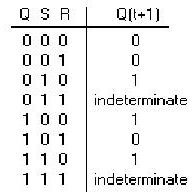From the above truth table we can deduce that:

Regardless of the value of R input, when the S input is 0 the value of present state Q is retained. Consider the first 2 rows of the above table.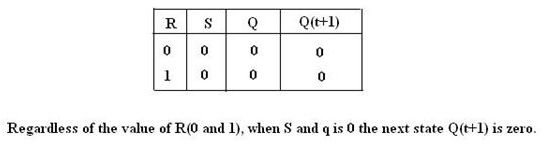Similarly consider the 5th row and 7th row of the RS truth table.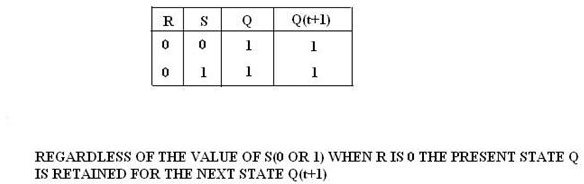Regardless of the value of S, When R is 0, the present state Q is retained for the next state Q (t+1).

From the above conclusions we can form the excitation table of RS flip-flop as shown below.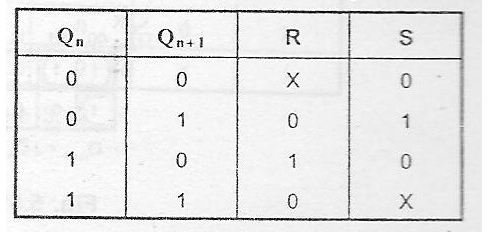In the above Figure, X represents don’t care condition (value has no significance). For example if X is found under R input, it indicates that the value of R will not affect the present or future (or) next state of the flip-flop.

From the above discussions we can conclude that, there are four possible transitions for a RS flip-flop.

The four transitions are:

0->0 transition; 0->1 transition, 1-> 0 transitions and 1 -> 1 transition.

## Exciataion and Truth Table: D Flip-Flop

Now let us discuss the Truth table and excitation table of D Flip-Flop.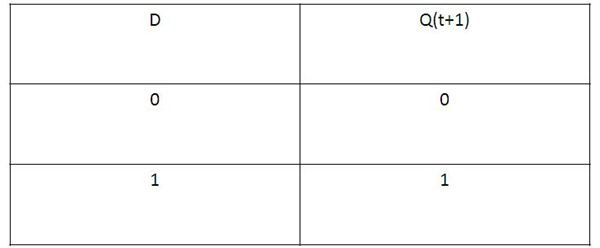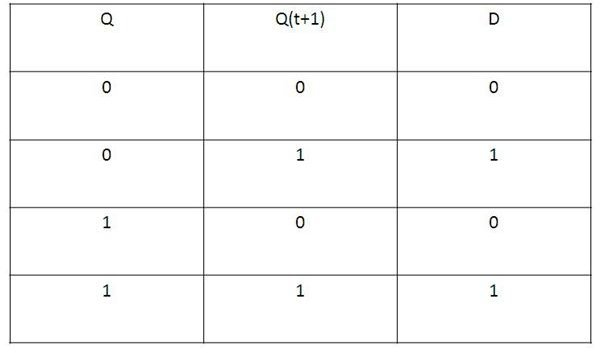From the above table it is clear that for a D flip-flop, the next state Q (t+1) is always equal to the D input. The next state Q (t+1) is independent of the present state Q.

From the K-map and excitation table we can say that the characteristic equation of D flip-flop is

Q (t+1) =D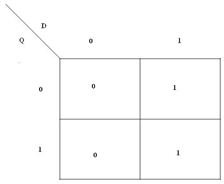## Converting an RS flip-flop To D Flip-flop

We can convert one flip-flop to another flip-flop by adding some additional gates to the existing flip-flop or giving some extra connections.

To convert a RS flip-flop into D flip-flop, we simply have to remap the state transitions of D flip-flop’s K-map into equations of R and S inputs of flip-flop.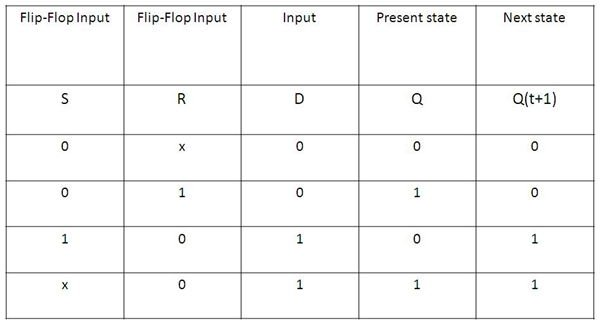The procedure for conversion of RS flip-flop to D flip-flop is as follows.

· Draw the K-map of R and S inputs separately with Input D and Present state Q.

· Fill the K-map using the data in the excitation table. When D=0 and Q=0, the next state Q (t+1) is 0. (Refer to D flip-flop truth table). For the next state to remain 0, the value of R and S should be X and 0 respectively. Here X denotes don’t care value. The value of R in this condition, will not affect the state of the system. 0->0 transition. R=X and S=0.

· When D=0 and Q=1, the value of Next state Q (t+1) should be 0. (Refer to D flip-flop truth table). Here there is a transition from 0 to 1. [Q=1 and Q (t+1) =0]. The RS input which corresponds to this transition is S=0 and R=1. 1-> 0 transition, R=1 and S=0.

· When D=1 and Q=0, the value of next state Q (t+1) should be 1(from D flip-flop truth table). Here there is 0 to 1 transition. The value of R and S should be 0 and 1 respectively. 0->1 transition, R=0 and S=1.

· When D=1 and Q=1, the value of next state should be equal to 1(from D flip-flop truth table). Here there is a 1->1 transition. The value of R and S corresponding to this transition is 0 and X respectively. X here represents don’t care condition. 1->1 transition, R=0 and S=X.

Thus from the above steps it is clear that the RS flip-flop can be converted to a D flip-flop.

K-Map and Logic diagram gives us a better understanding about the conversion of RS flip-flop to D flip-flop.

From the K-Map, we can conclude that S=D and R=D’.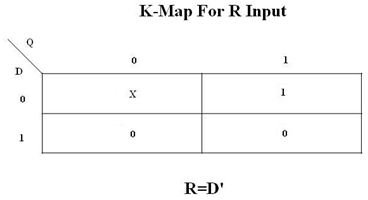## Logic Diagram

From the Logic diagram we can conclude that, S=D. D input is directly given to S input of RS flip-flop and Instead of R input, the complement of D value is given to R input.

Thus we can convert a RS flip-flop into a D flip-flop.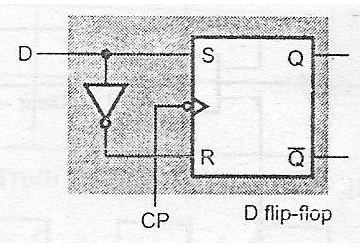In the Next article let’s discuss the other types of flip-flop conversions.